# DAV Class 6 Maths Chapter 5 Worksheet 3 Solutions

The DAV Class 6 Maths Solutions and DAV Class 6 Maths Chapter 5 Worksheet 3 Solutions of Percentage and its Applications offer comprehensive answers to textbook questions.

## DAV Class 6 Maths Ch 5 WS 3 Solutions

Question 1.
Find gain or loss percent.

(a) C.P. = ₹ 900, S.P. = ₹ 1080
Solution:
C.P. = ₹ 900, S.P. = ₹ 1080
Here, S.P. > C.P.
∴ Gain = S.P. – C.P.
= ₹ 1080 – ₹ 900 = ₹ 180
∴ Gains % = $$\frac{\text { Gain }}{\text { C.P. }}$$ × 100
== 20%
Hence, the required gain = 20%.(b) C.P. = ₹ 2400, S.P. = ₹ 2250
Solution:
C.P. = ₹ 2400,
S.P. = ₹ 2250
Here, S.P. < C.P.
∴ Loss = C.P. – S.P.
= ₹ 2400 – ₹ 2250
= ₹ 150
Loss % = $$\frac{\text { Loss }}{\text { C.P. }}$$ × 100
∴ Loss % == $$\frac{25}{4}$$ %
= 6 $$\frac{1}{4}$$ %
Hence, the required loss = 6 $$\frac{1}{4}$$ %.

(c) C.P. = ₹ 750, S.P. = ₹ 850
Solution:
C.P. = ₹ 750,
S.P. = ₹ 850 Here, S.P. > C.P.
∴ Gain = S.P. – C.P.
= ₹ 850 – ₹ 750 = ₹ 100
∴ Gain % = $$\frac{\text { Gain }}{\text { C.P. }}$$ × 100
=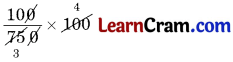= $$\frac{40}{3}$$ %
= 13 $$\frac{1}{3}$$%
Hence, the required gain

(d) C.P. = ₹ 10200, S.P. = ₹ 8670
Solution:
C.P. = ₹ 10200, S.P. = ₹ 8670
Here, S.P. < C.P.
∴ Loss = C.P. – S.P.
= ₹ 10200 – ₹ 8670 = ₹ 1530
Loss % = $$\frac{\text { Loss }}{\text { C.P. }}$$ × 100
∴ Loss % =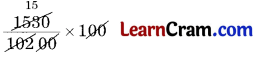= 15%
Hence, the required loss = 15%.Question 2.
A shopkeeper buys 30 chocolate bars at ₹ 15 each and sold all of them for ₹ 525. Find the profit percent he made. Solution:
C.P. of 1 chocolate bars = ₹ 15
C.P. of 30 chocolate bars = ₹ 15 × 30 = ₹ 450
S.P. of 30 chocolate bars = ₹ 525
Profit = S.P. – C.P.
= ₹ 525 – ₹ 450 = ₹ 75.
Profit percent = $$\frac{\text { Profit }}{\text { C.P. }}$$ × 100
=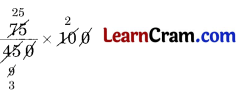= $$\frac{50}{3}$$ %
= 16$$\frac{2}{3}$$ %
Hence, the required profit = 16 $$\frac{2}{3}$$ %

Question 3.
A man buys a radio set for ₹ 900 and sells it for ₹ 972. What is his profit or loss percent?
Solution:
C.P. of radio set = ₹ 900
S.P. of the radio set = ₹ 972
Here, S.P. > C.P.
∴ Profit = S.P. – C.P.
= ₹ 972 – ₹ 900
= ₹ 72
∴ Profit percent = $$\frac{\text { Profit }}{\text { C.P. }}$$ × 100
=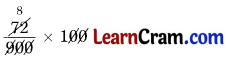= 8 %
Hence, the required profit = 8%.Question 4.
Mr. Gupta purchases a house for ₹ 2,50,000 and spends ₹ 50,000 on repairs. If he sells it for ₹ 4,00,000 find his gain percent.
Solution:
C.P. of house = ₹ 2,50,000
Money spent on repairs = ₹ 50,000
∴ Total C.P. = ₹ 2,50,000 + ₹ 50,000
= ₹ 3,00,000
S.P. of the house = ₹ 4,00,000
Gain = S.P. – C.P.
= ₹ 4,00,000 – ₹ 3,00,000
= ₹ 1,00,000
Gain percent = $$\frac{\text { Gain }}{\text { C.P. }}$$ × 100
=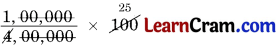= 25%
Hence, the required gain = 25%.